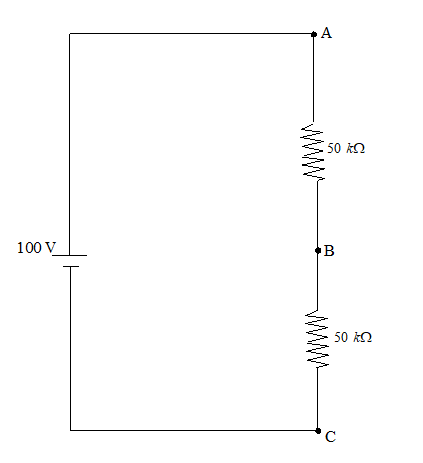In the adjacent shown circuit, a voltmeter of internal resistance R, when connected across B and C reads 1003 V . Neglecting the internal resistance of the battery, the value of R is (in kilo ohm)

# In the adjacent shown circuit, a voltmeter of internal resistance R, when connected across B and C reads $\frac{100}{3}\text{\hspace{0.17em}}V$ . Neglecting the internal resistance of the battery, the value of R is (in kilo ohm)Register to Get Free Mock Test and Study Material

+91

Verify OTP Code (required)

### Solution:

When a resistance of R is connected in parallel between B and C.

The effective resistance between B and C will be ${\mathrm{R}}_{\mathrm{BC}}=\frac{50.\mathrm{R}}{50+\mathrm{R}}$

So the potential difference between B and C is given by $\mathrm{V}\left(\frac{{\mathrm{R}}_{\mathrm{BC}}}{{\mathrm{R}}_{\mathrm{BC}}+{\mathrm{R}}_{\mathrm{AB}}}\right)$, which is given as $\frac{100}{3}\mathrm{V}$

$⇒\frac{{\mathrm{R}}_{\mathrm{BC}}}{{\mathrm{R}}_{\mathrm{BC}}+{\mathrm{R}}_{\mathrm{AB}}}=\frac{1}{3}$

on solving we get $\mathrm{R}=50\mathrm{\Omega }$Register to Get Free Mock Test and Study Material

+91

Verify OTP Code (required)